# Characteristic strip

(diff) ← Older revision | Latest revision (diff) | Newer revision → (diff)

of a first-order partial differential equation

A family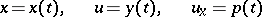of continuously-differentiable functions in an interval, satisfying the equations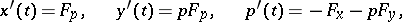where the multiplication of the vectors is the scalar product, and where(*)

is a non-linear first-order partial differential equation in the unknown function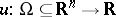. Here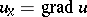,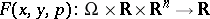,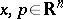,,.

The importance of a characteristic strip consists in the fact that it is used in the study of, and in the search for, solutions of equation (*).